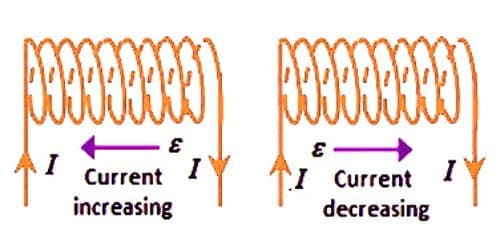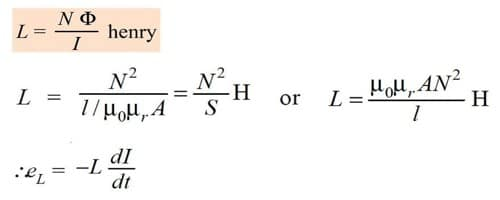# Explain Coefficient of Self Induction

Coefficient of self induction of the ratio of the electromotive force (emf) produced in a circuit by self-induction to the rate of modify of current producing it, articulated in henries. The property of a coil which enables to generate an opposing induced electromotive force (emf) in it when the current in the coil changes is called self induction.

Coefficient of self induction

When a current I flows through a coil, the magnetic flux (φ) linked with the coil is proportional to the current.

φ α I or φ = LI

where L is a constant of proportionality and is called coefficient of self induction of self-inductance.

If I = 1A, φ = L × 1, then L = φ Therefore, the coefficient of self-induction of a coil is numerically equal to the magnetic flux linked with a coil when unit current flows through it. According to laws of electromagnetic induction.

e = – dφ/dt = d/dt (LI)

or, e = – L (dI/dt)

If (dI/dt) = 1 A s-1, then L = − e

The coefficient of self induction of a coil is numerically equal to the opposing emf induced in the coil when the rate of change of current through the coil is unity. This property of inductor or coil due to which it opposes a change of current through it called the inductance denoted by letter L. The unit of self inductance is henry (H). 1 Henry is also equal to 1 Weber/ampere.One henry is defined as the self-inductance of a coil in which a change in the current of one ampere per second produces an opposing emf of one volt.

Explanation:

When we ascertain a current through an inductor or coil, it generates a magnetic field and this result in a magnetic flux transitory through the coil. An inductor consists of a coil wound on a core or former of an apposite material like solid or covered iron core or ferrites which are decidedly ferromagnetic substances.

If we differ the amount of current flowing in the coil with time, the magnetic flux connected with the coil also changes and an emf is induced in the coil. When the current through an inductor change is emf is induced in it which opposes this modify of current in the inductor. According to the Lenz’s law, the direction of induced emf is such that it opposes its cause i.e. it opposes the change in current or magnetic flux.

This incident of production of opposing induced emf in inductor or coil itself due to time-varying current in the coil is known as self induction.

In Summary –

• The value of the inductance will be high if the magnetic flux is stronger for the given value of current.
• The value of the Inductance also depends upon the material of the core and the number of turns in the coil or solenoid.
• The higher will be the value of the inductance in Henry, the rate of change of current will be lower.